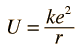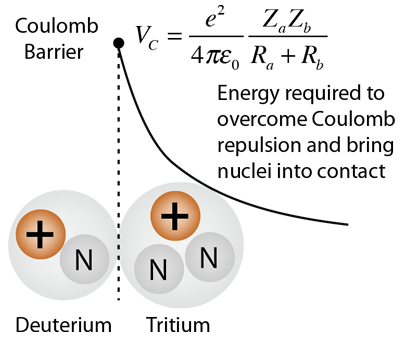# Coulomb Barrier for Fusion

In order to accomplish nuclear fusion, the particles involved must first overcome the electric repulsion to get close enough for the attractive nuclear strong force to take over to fuse the particles. This requires extremely high temperatures, if temperature alone is considered in the process. In the case of the proton cycle in stars, this barrier is penetrated by tunneling, allowing the process to proceed at lower temperatures than that which would be required at pressures attainable in the laboratory.

Considering the barrier to be the electric potential energy of two point charges (e.g., protons), the energy required to reach a separation r is given bywhere k = Coulomb's constant and e is the proton charge.

Given the radius r at which the nuclear attractive force becomes dominant, the temperature necessary to raise the average thermal energy to that point can be calculated.

 Calculation
Index

Fusion concepts

 HyperPhysics***** Nuclear R Nave
Go Back

# Calculation of Coulomb BarrierThe height of the Coulomb barrier can be calculated if the nuclear separation and the charges of the particles are known. The nuclear radii can be calculated from the mass numbers A and atomic numbers Z. Note that this models a nucleus as a sphere of constant charge density.
For Aa = and Za = , Ra = x10^ m = fermi.

For Ab = and Zb = , Rb = x10^ m = fermi.

The height of the Coulomb Barrier is

VC = x 10^J = x 10^eV = keV = MeV.

The temperature required to provide this energy as an average thermal energy for each particle would be

Kinetic temperature T = x 10^ K

Of course for head-on collisions between particles only half of that energy would be required of each particle, so you could cut that temperature in half. The above temperature is calculated as a reference value.

 Discussion of Coulomb barrier Why is this temperature too high?
Index

Fusion concepts

References:
Krane,
Sec 14.2

 HyperPhysics***** Nuclear R Nave
Go Back

# Critical Ignition Temperature for Fusion

The fusion temperature obtained by setting the average thermal energy equal to the coulomb barrier gives too high a temperature because fusion can be initiated by those particles which are out on the high-energy tail of the Maxwellian distribution of particle energies. The critical ignition temperature is lowered further by the fact that some particles which have energies below the coulomb barrier can tunnel through the barrier.

The presumed height of the coulomb barrier is based upon the distance at which the nuclear strong force could overcome the coulomb repulsion. The required temperature may be overestimated if the classical radii of the nuclei are used for this distance, since the range of the strong interaction is significantly greater than a classical proton radius.

When trying to model the probability of nuclear fusion, the typical approach is to model it as a "cross-section" for the reaction to occur. This approach is perhaps more apparent in evaluating particle scattering like Rutherford scattering, but the language is often used for nuclear fusion as well. For the purposes here, cross-section can be taken to mean the probability for nuclear fusion to occur. Modeling this cross-section involves taking into account the probability for tunneling through the coulomb barrier. This probability is higher for higher energy particles, but because of the Maxwellian distribution, there are fewer of these high energy particles. Also, the effective energy of collision between the particles for fusion depends on their relative velocities, so the model calculation for nuclear fusion yield involves averaging over all relative velocities. The results of such modeling are presented as a plot of fusion cross-section as a function of average particle energy.With all these considerations, the energy requirements for the nuclear fusion of deuterium- tritium and for deuterium-deuterium are dramatically lower than the coulomb barrier, but they are nevertheless very difficult to attain in a controlled manner. The references suggest that the currently attainable particle energies in thermonuclear reactors is in the range 1 - 10 keV. If you just substitute those energies into the thermal energy relationship, then 1keV corresponds to a temperature of 0.77 x 107K and 10 keV corresponds to 0.77 x 108K.

The TFTR reached a temperature of 5.1 x 108 K, well above the critical ignition temperature for D-T fusion.

References:

Index

Fusion concepts

References:
Krane,
Sec 14.2

 HyperPhysics***** Nuclear R Nave
Go Back

# Temperatures for Fusion

The temperatures required to overcome the coulomb barrier for fusion to occur are so high as to require extraordinary means for their achievement. Such thermally initiated reactions are commonly called thermonuclear fusion. With particle energies in the range of 1-10keV, the temperatures are in the range 107-108K.

In the sun, the proton-proton cycle of fusion is presumed to proceed at a much lower temperature because of the extremely high density and high population of particles.

 Interior of the sun, proton cycle: 1.5 x 107 KThe most feasible near-term options for thermonuclear fission appear to be deuterium-tritium, deuterium-deuterium, and deuterium-helium 3. To obtain an approximate temperature for any location on the diagram, use the corresponding energy in the relationship for thermal energy.

References:

Index

Fusion concepts

References:
Krane,
Sec 14.2

 HyperPhysics***** Nuclear R Nave
Go Back